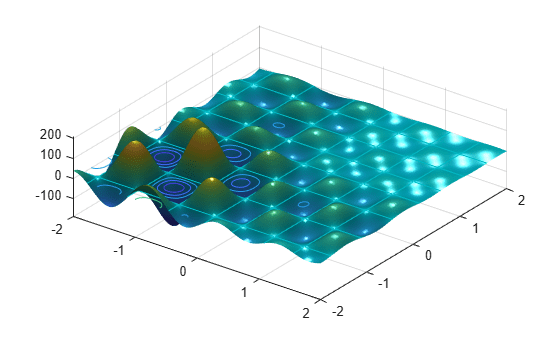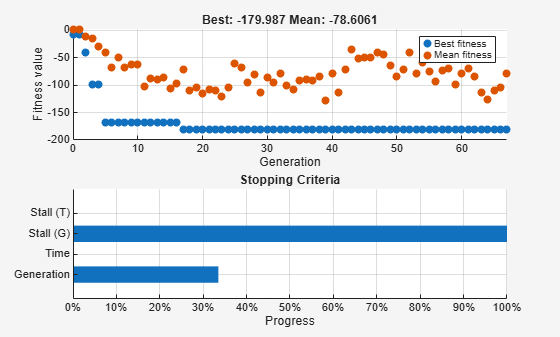# Effects of Genetic Algorithm Options

This example shows the effects of some options for the genetic algorithm function `ga`. You create and change options by using the `optimoptions` function.

### Setting Up a Problem for `ga`

`ga` searches for a minimum of a function using the genetic algorithm. For this example, use `ga` to minimize the fitness function `shufcn`, a real valued function of two variables.

Pot `shufcn` over the range `= [-2 2;-2 2]` by calling `plotobjective`.

`plotobjective(@shufcn,[-2 2; -2 2]);`To use the `ga` solver, provide at least two input arguments, a fitness function and the number of variables in the problem. The first two output arguments returned by `ga` are `x`, the best point found, and `Fval`, the function value at the best point. A third output argument, `exitFlag` tells you the reason why `ga` stopped. `ga` can also return a fourth argument, `Output`, which contains information about the performance of the solver.

```FitnessFunction = @shufcn; numberOfVariables = 2;```

Run the `ga` solver.

```rng default % For reproducibility [x,Fval,exitFlag,Output] = ga(FitnessFunction,numberOfVariables);```
```Optimization terminated: average change in the fitness value less than options.FunctionTolerance. ```
`fprintf('The number of generations was : %d\n', Output.generations);`
```The number of generations was : 124 ```
`fprintf('The number of function evaluations was : %d\n', Output.funccount);`
```The number of function evaluations was : 5881 ```
`fprintf('The best function value found was : %g\n', Fval);`
```The best function value found was : -186.199 ```

If you run this example without the `rng default` command, your result can differ, because `ga` is a stochastic algorithm.

### How the Genetic Algorithm Works

The Genetic Algorithm works on a population using a set of operators that are applied to the population. A population is a set of points in the design space. The initial population is generated randomly by default. The next generation of the population is computed using the fitness of the individuals in the current generation. For details, see How the Genetic Algorithm Works

To visualize the solver performance while running, set a `'PlotFcn'` option using `optimoptions`. In this case, select two plot functions in a cell array. Set `gaplotbestf`, which plots the best and mean score of the population at every generation. Also set `gaplotstopping`, which plots the percentage of stopping criteria satisfied.

`opts = optimoptions(@ga,'PlotFcn',{@gaplotbestf,@gaplotstopping});`

Run the `ga` solver, including the `opts` argument.

```[x,Fval,exitFlag,Output] = ... ga(FitnessFunction,numberOfVariables,[],[],[],[],[],[],[],opts);```
```Optimization terminated: average change in the fitness value less than options.FunctionTolerance. ```### Specify Population Options

Specify Population Size

`ga` creates a default initial population by using a uniform random number generator. The default population size used by `ga` is 50 when the number of decision variables is less than 5 and 200 otherwise. This size can be a poor one for some problems; a smaller population size can be sufficient for smaller problems. Since the current problem has only two variables, specify a population size of 10. Set the value of the option `PopulationSize` to 10 in the existing options, `opts`.

`opts.PopulationSize = 10;`

Specify Initial Population Range

The default method for generating an initial population uses a uniform random number generator. For problems without integer constraints, `ga` creates an initial population where all the points are in the range –10 to 10. For example, a population of size 3 in a problem with two variables could look like:

`Population = [-10,-10] + 20*rand(3,2);`

The initial range can be set by changing the `InitialPopulationRange` option. The range must be a matrix with two rows. If the range has only one column, i.e., it is 2-by-1, then the range of every variable is the given range. For example, if you set the range to `[-1; 1]`, then the initial range for both variables is –1 to 1. To specify a different initial range for each variable, the range must be specified as a matrix with two rows and `numberOfVariables` columns. For example if you set the range to `[-1 0; 1 2]`, then the first variable has range –1 to 1, and the second variable has range 0 to 2 (each column corresponds to a variable).

Modify the value of the option `InitialPopulationRange` in the existing options, `opts`.

`opts.InitialPopulationRange = [-1 0; 1 2];`

Run the `ga` solver.

```[x,Fval,exitFlag,Output] = ga(FitnessFunction,numberOfVariables,[],[],[], ... [],[],[],[],opts);```
```Optimization terminated: average change in the fitness value less than options.FunctionTolerance. ````fprintf('The number of generations was : %d\n', Output.generations);`
```The number of generations was : 67 ```
`fprintf('The number of function evaluations was : %d\n', Output.funccount);`
```The number of function evaluations was : 614 ```
`fprintf('The best function value found was : %g\n', Fval);`
```The best function value found was : -179.987 ```

### Reproduce Results

By default, `ga` starts with a random initial population which is created using MATLAB® random number generators. The next generation is produced using `ga` operators that also use these same random number generators. Every time a random number is generated, the state of the random number generators change. This means that even if you do not change any options, when you run again you can get different results.

Run the solver twice to show this phenomenon.

Run the `ga` solver.

`[x,Fval,exitFlag,Output] = ga(FitnessFunction,numberOfVariables);`
```Optimization terminated: average change in the fitness value less than options.FunctionTolerance. ```
`fprintf('The best function value found was : %g\n', Fval);`
```The best function value found was : -186.484 ```

Run `ga` again.

`[x,Fval,exitFlag,Output] = ga(FitnessFunction,numberOfVariables);`
```Optimization terminated: average change in the fitness value less than options.FunctionTolerance. ```
`fprintf('The best function value found was : %g\n', Fval);`
```The best function value found was : -185.867 ```

In the previous two runs, `ga` gave different results. The results are different because the states of the random number generators have changed from one run to another.

If you know that you want to reproduce your results before you run `ga`, you can save the state of the random number stream.

`thestate = rng;`

Run `ga`.

`[x,Fval,exitFlag,Output] = ga(FitnessFunction,numberOfVariables);`
```Optimization terminated: average change in the fitness value less than options.FunctionTolerance. ```
`fprintf('The best function value found was : %g\n', Fval);`
```The best function value found was : -186.467 ```

Reset the stream and rerun `ga`. The results are identical to the previous run.

```rng(thestate); [x,Fval,exitFlag,Output] = ga(FitnessFunction,numberOfVariables);```
```Optimization terminated: average change in the fitness value less than options.FunctionTolerance. ```
`fprintf('The best function value found was : %g\n', Fval);`
```The best function value found was : -186.467 ```

However, you might not have realized that you would want to try to reproduce the results before running `ga`. In that case, as long as you have the `output` structure, you can reset the random number generator as follows.

```strm = RandStream.getGlobalStream; strm.State = Output.rngstate.State;```

Rerun `ga`. Again, the results are identical.

`[x,Fval,exitFlag,Output] = ga(FitnessFunction,numberOfVariables);`
```Optimization terminated: average change in the fitness value less than options.FunctionTolerance. ```
`fprintf('The best function value found was : %g\n', Fval);`
```The best function value found was : -186.467 ```

### Modify Stopping Criteria

`ga` uses four different criteria to determine when to stop the solver. `ga` stops when the maximum number of generations is reached; by default this number is 100 times the number of variables. `ga` also detects if there is no change in the best fitness value for some time given in seconds (stall time limit), or for some number of generations (maximum stall generations). Another criteria is the maximum time limit in seconds. Modify the stopping criteria to increase the maximum number of generations to 300 and the maximum stall generations to 100.

`opts = optimoptions(opts,'MaxGenerations',300,'MaxStallGenerations', 100);`

Rerun the `ga` solver.

```[x,Fval,exitFlag,Output] = ga(FitnessFunction,numberOfVariables,[],[],[], ... [],[],[],[],opts);```
```Optimization terminated: average change in the fitness value less than options.FunctionTolerance. ````fprintf('The number of generations was : %d\n', Output.generations);`
```The number of generations was : 299 ```
`fprintf('The number of function evaluations was : %d\n', Output.funccount);`
```The number of function evaluations was : 2702 ```
`fprintf('The best function value found was : %g\n', Fval);`
```The best function value found was : -186.729 ```

### Choose `ga` Operators

`ga` starts with a random set of points in the population and uses operators to produce the next generation of the population. The different operators are scaling, selection, crossover, and mutation. The toolbox provides several functions to choose from for each operator. Choose `fitscalingprop` for `FitnessScalingFcn` and `selectiontournament` for `SelectionFcn`.

```opts = optimoptions(@ga,'SelectionFcn',@selectiontournament, ... 'FitnessScalingFcn',@fitscalingprop);```

Rerun `ga`.

```[x,Fval,exitFlag,Output] = ga(FitnessFunction,numberOfVariables,[],[],[], ... [],[],[],[],opts);```
```Optimization terminated: average change in the fitness value less than options.FunctionTolerance. ```
`fprintf('The number of generations was : %d\n', Output.generations);`
```The number of generations was : 52 ```
`fprintf('The number of function evaluations was : %d\n', Output.funccount);`
```The number of function evaluations was : 2497 ```
`fprintf('The best function value found was : %g\n', Fval);`
```The best function value found was : -186.417 ```

The best function value can improve or get worse by choosing different operators. Choosing a good set of operators for your problem is often best done by experimentation.

Watch now